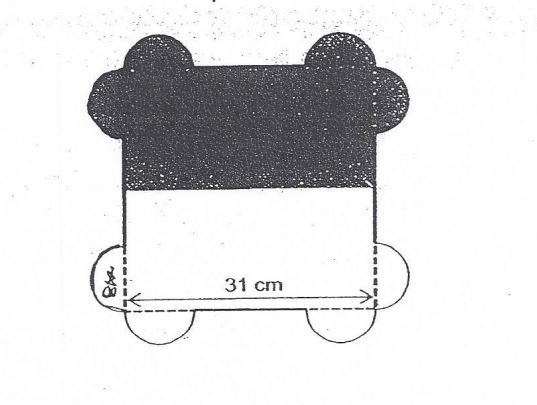# Question 1 of 84

The table mat below is made up of identical semi – circles and a 31-cm square.
(a) Find the perimeter of the entire table mat.
(b) Half of the table mat shaded. Find the area of the shaded part. $(Take \pi = \frac{22}{7})$A
(a) 152cm
(b) 553.5cm
B
(a) 154cm
(b) 555.5cm
C
(a) 156cm
(b) 557.5cm
D
(a) 158cm
(b) 559.5cm
E
None of the above